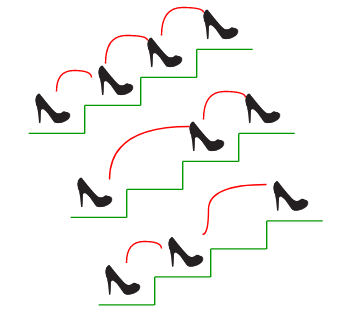# Python Program to Count ways to reach the n’th stair

There are n stairs, a person standing at the bottom wants to reach the top. The person can climb either 1 stair or 2 stairs at a time. Count the number of ways, the person can reach the top.Consider the example shown in diagram. The value of n is 3. There are 3 ways to reach the top. The diagram is taken from Easier Fibonacci puzzles

## Python

 `# A program to count the number of ways to reach n'th stair ` ` `  `# Recurssive program to find n'th fibonacci number ` `def` `fib(n): ` `    ``if` `n <``=` `1``: ` `        ``return` `n ` `    ``return` `fib(n``-``1``) ``+` `fib(n``-``2``) ` ` `  `# returns no. of ways to reach s'th stair ` `def` `countWays(s): ` `    ``return` `fib(s ``+` `1``) ` ` `  `# Driver program ` ` `  `s ``=` `4` `print` `"Number of ways = "``, ` `print` `countWays(s) ` ` `  `# Contributed by Harshit Agrawal `

Output:

```Number of ways =  5
```

The time complexity of the above implementation is exponential (golden ratio raised to power n). It can be optimized to work in O(Logn) time using the previously discussed Fibonacci function optimizations.

## Python

 `# A program to count the number of ways to reach n'th stair ` ` `  `# Recursive function used by countWays ` `def` `countWaysUtil(n, m): ` `    ``if` `n <``=` `1``: ` `        ``return` `n ` `    ``res ``=` `0` `    ``i ``=` `1` `    ``while` `i<``=` `m ``and` `i<``=` `n: ` `        ``res ``=` `res ``+` `countWaysUtil(n``-``i, m) ` `        ``i ``=` `i ``+` `1` `    ``return` `res ` `     `  `# Returns number of ways to reach s'th stair     ` `def` `countWays(s, m): ` `    ``return` `countWaysUtil(s ``+` `1``, m) ` `     `  ` `  `# Driver program ` `s, m ``=` `4``, ``2` `print` `"Number of ways ="``, countWays(s, m) ` ` `  `# Contributed by Harshit Agrawal `

Output:

```Number of ways = 5
```

## Python

 `# A program to count the number of ways to reach n'th stair ` ` `  `# Recursive function used by countWays ` `def` `countWaysUtil(n, m): ` `    ``res ``=` `[``0` `for` `x ``in` `range``(n)] ``# Creates list res witth all elements 0 ` `    ``res[``0``], res[``1``] ``=` `1``, ``1` `     `  `    ``for` `i ``in` `range``(``2``, n): ` `        ``j ``=` `1` `        ``while` `j<``=` `m ``and` `j<``=` `i: ` `            ``res[i] ``=` `res[i] ``+` `res[i``-``j] ` `            ``j ``=` `j ``+` `1`  `    ``return` `res[n``-``1``] ` ` `  `# Returns number of ways to reach s'th stair ` `def` `countWays(s, m): ` `    ``return` `countWaysUtil(s ``+` `1``, m) ` `     `  `# Driver Program ` `s, m ``=` `4``, ``2` `print` `"Number of ways ="``, countWays(s, m) ` `     `  `# Contributed by Harshit Agrawal `

Output:

```Number of ways = 5
```

Please refer complete article on Count ways to reach the n’th stair for more details!

My Personal Notes arrow_drop_up

Improved By : Akanksha_Rai

Article Tags :

Be the First to upvote.

Please write to us at contribute@geeksforgeeks.org to report any issue with the above content.